# Original question is above, and is for C programming. As you will see in my code...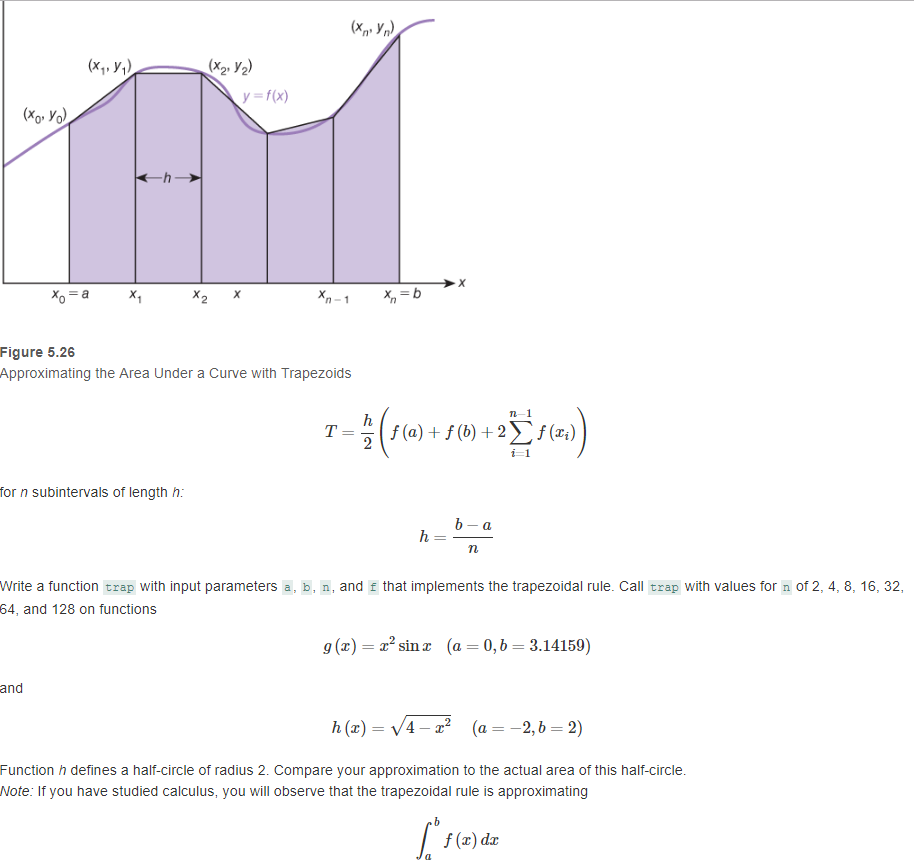Original question is above, and is for C programming. As you will see in my code below, I am not sure how to properly implement the functions I made for g(x) and h(x) (I call them g_x and h_x) into my function called trap. I know that in my main function in my function call to trap, I have to put something in the parentheses of g_x() and h_x() since they both take a type double argument, however I'm not sure what to write since ultimately the arguments they take will actually come from the function trap

/*
Create a function "trap" with input parameters a, b, n, and f that implements the trapezoidal rule
to approximate the integral of f(x) from a to b. Call trap with values for n of 2, 4, 8, 16, 32, 64, 128
on functions g(x) = x^2sinxx (a = 0, b = PI) and h(x) = sqrt(4-x^2) (a = -2, b = 2)
*/

#include
#include
#define PI 3.14159
double g_x(double x);
double h_x(double x);
double trap(int n, double a, double b, double f(double));
int main(int argc, char *argv[]) {
int n;
printf("TRAPEZOIDAL RULE g(x) = x^2sin(x), a = 0, b = PI\n");
for (n = 2; n <= 128; n *= 2)
{
printf("for n = %d, area is approximately %.4f\n", n, trap(n, 0, PI, g_x()));
}
printf("\n");
printf("TRAPEZOIDAL RULE h(x) = sqrt(4 - x^2), a = -2, b = 2\n");
for (n = 2; n <= 128; n *= 2)
{
printf("for n = %d, area is approximately %.4f\n", n, trap(n, -2, 2, h_x()));
}
return 0;
}

double trap(int n, double a, double b, double f(double))
{
double h = (b - a) / n;
double riemann_sum = 0;
double area_approximation;
double i;
for (i = a + h; i <= b - h; i += h)
{
riemann_sum += f(i);
}
area_approximation = (h / 2) * (f(a) + f(b) + 2 * riemann_sum);
return area_approximation;
}

double g_x(double x)
{
return pow(x, 2) * sin(x);
}
double h_x(double x)
{
return sqrt(4 - pow(x, 2));
}

nt y y- fox) (xo Yo) Figure 5.26 Approximating the Area Under a Curve with Trapezoids n-1 T(a)(ai) for n subintervals of length h Write a function trap with input parameters a, b , and f that implements the trapezoidal rule. Call trap with values for n of 2, 4, 8, 16, 32 64, and 128 on functions g (x)sinz (a - 0,b 3.14159) and h (x)- V4-z' (a2, b-2) Function h defines a half-circle of radius 2. Compare your approximation to the actual area of this half-circle Note: If you have studied calculus, you will observe that the trapezoidal rule is approximating f (z) dr

THE PROBLEM IN YOUR CODE IS THAT WHEN YOU CALL THE METHOD TRAP, AND PASSING ANOTHER FUNCTION CALL AS PARAMETER WHICH WILL SIMPLY MEAN THAT FIRST THE FUNCTION g_x() OR h_x() WILL BE CALLED.

OR

ANOTHER METHOD CAN'T BE PASSED AS PARAMETER IN A METHOD AS THIS.

IN THIS CASE THE PARAMETER THAT WILL BE PASSED WILL BE THE RESULT OF THAT ANOTHER METHOD.

THUS, JUST PASS SOME char DATA WHICH WILL BE USED TO IDENTIFY WILL FUNCTION SHOULD BE CALLED AND USE IF-ELSE CONDITION.

THE CODE IS AS FOLLOWS-

#include<stdio.h>
#include<math.h>
#define PI 3.14159

double g_x(double x);
double h_x(double x);
double trap(int n, double a, double b, char F);

int main(int argc, char *argv[])
{
int n;
printf("TRAPEZOIDAL RULE g(x) = x^2sin(x), a = 0, b = PI\n");
for (n = 2; n <= 128; n *= 2)
{
printf("for n = %d, area is approximately %.4f\n", n, trap(n, 0, PI, 'g'));
}
printf("\n");

printf("TRAPEZOIDAL RULE h(x) = sqrt(4 - x^2), a = -2, b = 2\n");
for (n = 2; n <= 128; n *= 2)
{
printf("for n = %d, area is approximately %.4f\n", n, trap(n, -2, 2, 'h'));
}
return 0;
}

double trap(int n, double a, double b, char F)
{
double h = (b - a) / n;
double riemann_sum = 0;
double area_approximation;
double i;
if(F=='g')
{
for (i = a + h; i <= b - h; i += h)
riemann_sum += g_x(i);
area_approximation = (h / 2) * (g_x(a) + g_x(b) + 2 * riemann_sum);
return area_approximation;
}

else
{
for (i = a + h; i <= b - h; i += h)
riemann_sum += h_x(i);
area_approximation = (h / 2) * (h_x(a) + h_x(b) + 2 * riemann_sum);
return area_approximation;
}
}

double g_x(double x)
{
return pow(x, 2) * sin(x);
}

double h_x(double x)
{
return sqrt(4 - pow(x, 2));
}

#### Earn Coin

Coins can be redeemed for fabulous gifts.

Similar Homework Help Questions
• ### So I have a question in regards to my program. I'm learning to program in C...

So I have a question in regards to my program. I'm learning to program in C and I was curious about the use of functions. We don't get into them in this class but I wanted to see how they work. Here is my program and I would like to create a function for each of my menu options. I created a function to display and read the menu and the option that is entered, but I would like to...

• ### NEED CODE HELPS WITH C PROGRAMMING. ISSUES EXPLAINED IN BOLD. COMPARING TWO LETTERS AND CALLING A...

NEED CODE HELPS WITH C PROGRAMMING. ISSUES EXPLAINED IN BOLD. COMPARING TWO LETTERS AND CALLING A FUNCTION.   Problem are explained in bold #include <stdio.h> #include <string.h> #include <stdlib.h> #define pi 3.1415 void Area (double n); void VolSphere (double n); void Circumference (double n); void AllCalc (); // i dont know if the declaration of the function is correct AllCalc = AllCalculations //function main begins program execution int main (void) { puts("-Calculating Circle Circumference, Circle Area or Sphere Volume-\n"); void (*f)...

• ### Cant find what is wrong to fix the code. can you please fix the code. The...

Cant find what is wrong to fix the code. can you please fix the code. The function is: sin^-1 (x/5) #include <iostream> #include <iomanip> #include <math.h> #include <cmath> using namespace std; double f(double x) { return pow(sin, -1)*(x / 5); } int main() { int i, n; double a = 0.0, b =1.0, h, s = 0.0, x, xbar; double s1, s2, s3; cout << " Enter # of partitions n "; cin >> n; cout << " n =...

• ### C++ Could you check my code, it work but professor said that there are some mistakes(check...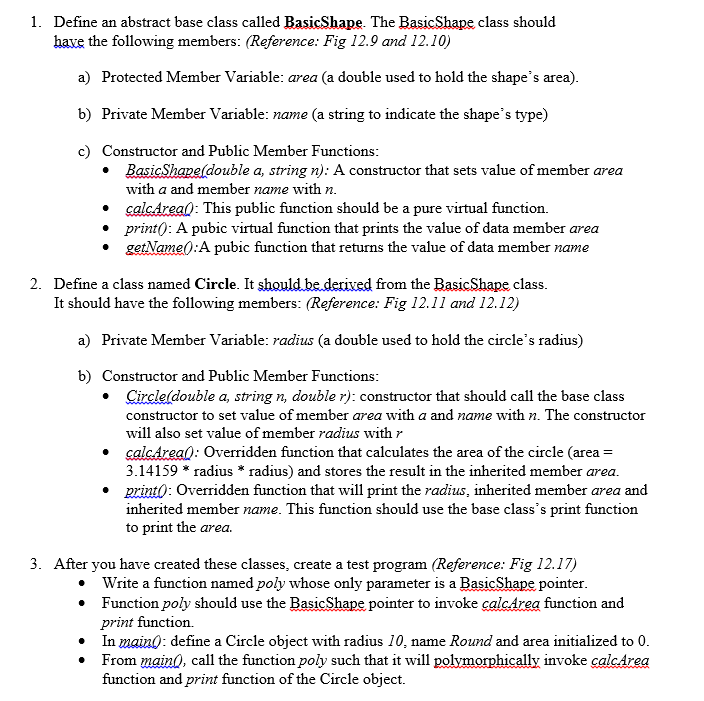C++ Could you check my code, it work but professor said that there are some mistakes(check virtual functions, prin() and others, according assignment) . Assignment: My code: #include<iostream> #include<string> using namespace std; class BasicShape { //Abstract base class protected: double area; private:    string name; public: BasicShape(double a, string n) { area=a; name=n; } void virtual calcArea()=0;//Pure Virtual Function virtual void print() { cout<<"Area of "<<getName()<<" is: "<<area<<"\n"; } string getName(){    return name; } }; class Circle : public...

• ### C Programming - RSA encryption Hi there, I'm struggling with writing a C program to encrypt and decrypt a string usi...

C Programming - RSA encryption Hi there, I'm struggling with writing a C program to encrypt and decrypt a string using the RSA algorithm (C90 language only). It can contain ONLY the following libraries: stdio, stdlib, math and string. I want to use the following function prototypes to try and implement things: /*Check whether a given number is prime or not*/ int checkPrime(int n) /*to find gcd*/ int gcd(int a, int h) void Encrypt(); void Decrypt(); int getPublicKeys(); int getPrivateKeys();...

• ### I wrote a program which computes the area and perimeter of a square, circle, or rectangle. As you will see in my main function, there is a for loop in which the user is supposed to be able repeat the...

I wrote a program which computes the area and perimeter of a square, circle, or rectangle. As you will see in my main function, there is a for loop in which the user is supposed to be able repeat the program until they enter "q" to quit. However, my program runs through one time, the prompt appears again, but then it terminates before the user is allowed to respond to the prompt again. I'm not able to debug why this...

• ### c++ Please help! i keep getting an error message. I think my main problem is not...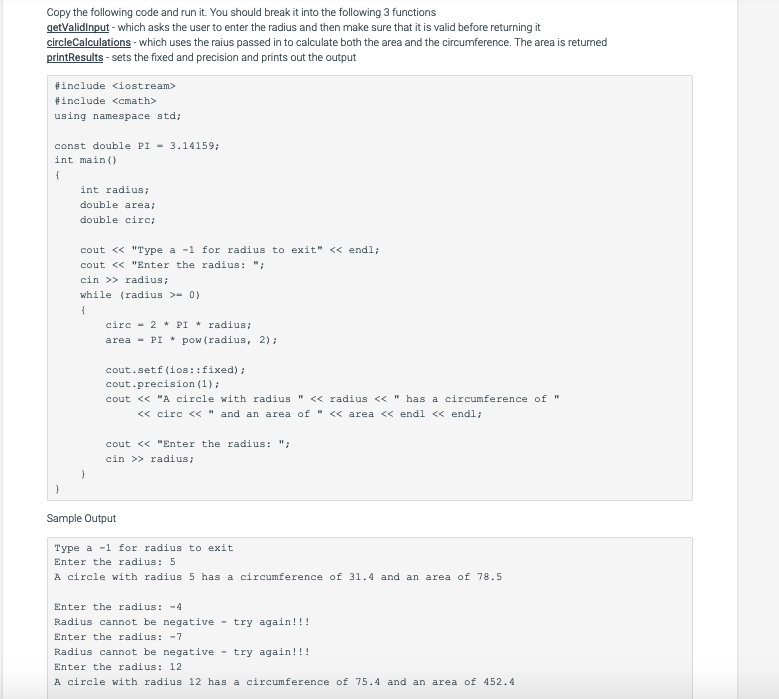c++ Please help! i keep getting an error message. I think my main problem is not understanding the circle calculations function and how both the area and the circumference is returned from the function. I know & needs to be used, but I am unsure how to use it. Copy the following code and run it. You should break it into the following 3 functions getValidinput - which asks the user to enter the radius and then make sure that...

• ### #include <stdio.h> .. int main(int argc, char *argv[]) { int base, power; printf("enter base and power:...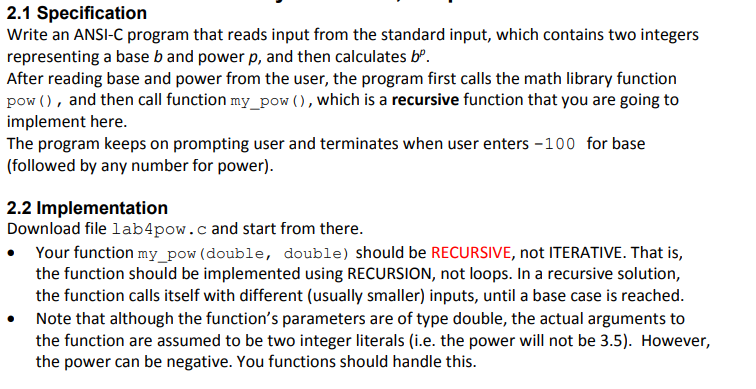#include <stdio.h> .. int main(int argc, char *argv[]) { int base, power; printf("enter base and power: "); scanf("%d %d", &base, &power); while (base != -100){ double res = pow(base, power); double res2 = my_pow(base, power); printf("pow: %.4f\n", res); printf("my_pow: %.4f\n", res2); .... } return 0; } // this function should be RECURSIVE // should not use any loop here double my_pow(double base, double p) { } lab4pow.c file contains: 2.1 Specification Write an ANSI-C program that reads input from the...

• ### 1. Simpson's rule. Simpson's rule is a different formula for numerical integration of lºf (d.x which...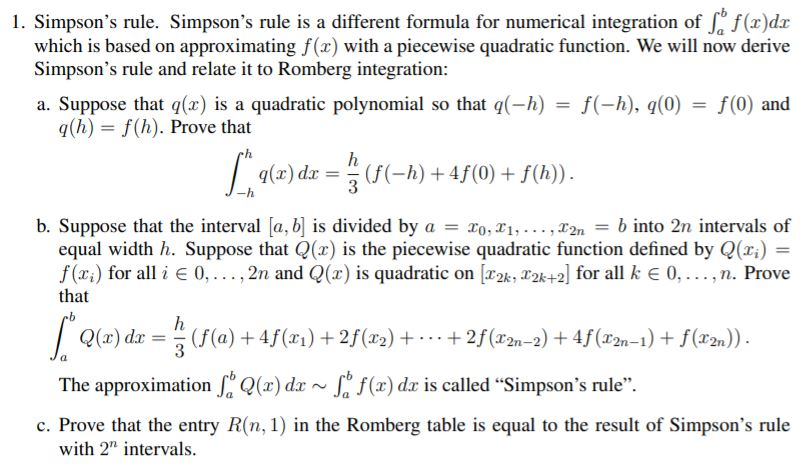1. Simpson's rule. Simpson's rule is a different formula for numerical integration of lºf (d.x which is based on approximating f(2) with a piecewise quadratic function. We will now derive Simpson's rule and relate it to Romberg integration: a. Suppose that (2) is a quadratic polynomial so that q(-h) = f(-h), q0) = f(0) and q(h) = f(h). Prove that 92 f(-h) + 4f(0) + f(h)). -h b. Suppose that the interval [a, b] is divided by a = 20,...

• ### C Programming Question Hi, I have the following code and in my submission I'm supposed to...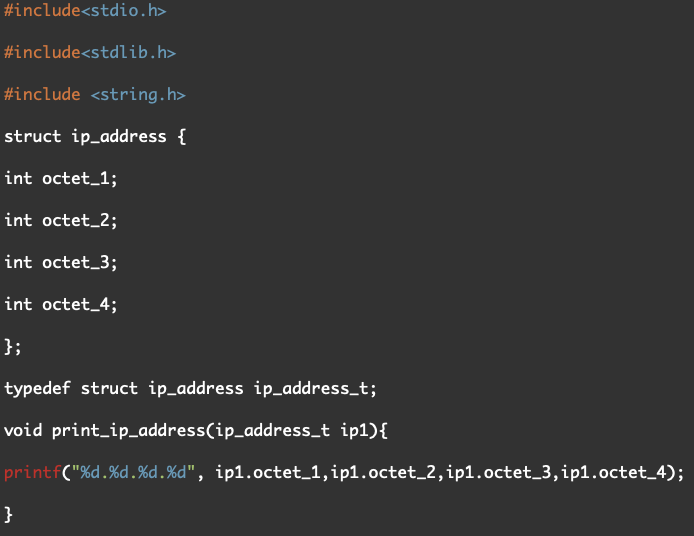C Programming Question Hi, I have the following code and in my submission I'm supposed to only include function definitions (i.e. implementations) in the file. However, I'm not NOT required to write the main, struct definitions and function prototypes. Could someone help me fix this code? Text Version: #include<stdio.h> #include<stdlib.h> #include <string.h> struct ip_address { int octet_1; int octet_2; int octet_3; int octet_4; }; typedef struct ip_address ip_address_t; void print_ip_address(ip_address_t ip1){ printf("%d.%d.%d.%d", ip1.octet_1,ip1.octet_2,ip1.octet_3,ip1.octet_4); } int is_valid(ip_address_t ip1){ if(ip1.octet_1 < 0...